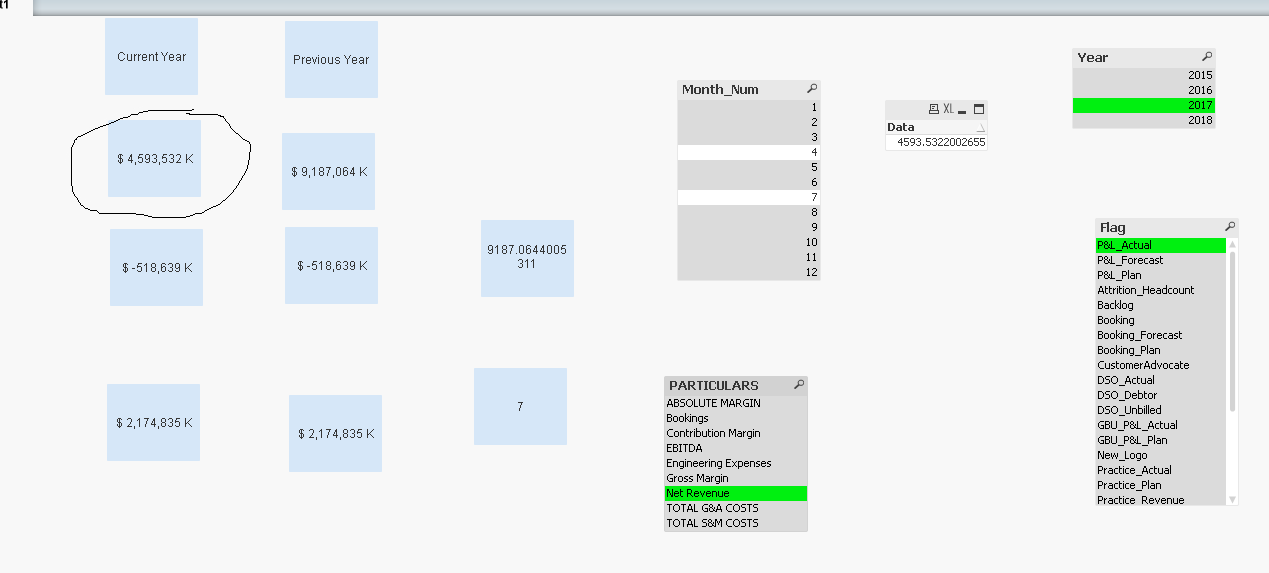# QlikView App Dev

Discussion Board for collaboration related to QlikView App Development.

Announcements
Join this live chat April 6, 10AM EST - QlikView to Qlik Sense REGISTER
cancel
Showing results for
Did you mean:Specialist

## Set analysis Syntax not working properly for current year and PY

Hi All,

PFA.

in text boxes I have shown it seems results are not showing properly for current year and Previous year.

We  need to show the results for max year and max year - 1 for max month.

Thanks

1 Solution

Accepted SolutionsSpecialist

The in that case this is your corrected expression for current Year

=if((Sum({<Year={'\$(=(max(Year)))'},Month_Num={"\$(=max(Month_Num))"},Flag={'P&L_Actual'},PARTICULARS={'Net Revenue'}>}Data))

=0,

(Num(Sum({<Year={'\$(=(max(Year)-1))'},Month_Num={"\$(=max(Month_Num))"},Flag={'P&L_Actual'},PARTICULARS={'Net Revenue'}>}Data)*1000,'\$ #,##0 K')),

(Num(Sum({<Year={'\$(=(max(Year)))'},Month_Num={"\$(=max(Month_Num))"},Flag={'P&L_Actual'},PARTICULARS={'Net Revenue'}>}Data)*1000,'\$ #,##0 K'))).

check the screenshot

Regards,

14 RepliesNot applicable

Hi,

Syntax error is there

Correct syntax is

sum({<DOJ={\$(=Max(Year)),\$(=max(year-1))}>}sal)Partner

Looks ok to me. Where exactly you are not seeing the data or not able to match to the source.Specialist

Loooks ok to me as you do not have data for 2018 it returns the correct result for 2017 .

Where do u find the problem .Specialist
Author

Hi,

It seems it is not showing the results for maximum  month number.

Regards,

DeepakMaster

Hi,

if you Select what you have in Your Expression, you have this:Your Expression is also a little bit strange if you only what to see previous and current max(year).

Can you tell us more what you expect?Specialist

The maximum month number for the the maximum year 2018 is April which does not have corresponding values in the Data field hence u are unable to see the values.

regards,Specialist
Author

Hi,

For example

For 2017 max month is july so only data for july need to show.

For 2016 data only for Apr.

ThanksSpecialist

The in that case this is your corrected expression for current Year

=if((Sum({<Year={'\$(=(max(Year)))'},Month_Num={"\$(=max(Month_Num))"},Flag={'P&L_Actual'},PARTICULARS={'Net Revenue'}>}Data))

=0,

(Num(Sum({<Year={'\$(=(max(Year)-1))'},Month_Num={"\$(=max(Month_Num))"},Flag={'P&L_Actual'},PARTICULARS={'Net Revenue'}>}Data)*1000,'\$ #,##0 K')),

(Num(Sum({<Year={'\$(=(max(Year)))'},Month_Num={"\$(=max(Month_Num))"},Flag={'P&L_Actual'},PARTICULARS={'Net Revenue'}>}Data)*1000,'\$ #,##0 K'))).

check the screenshot

Regards,